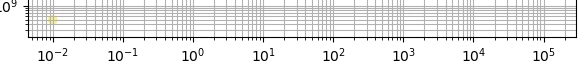# How to do x_axis = log(log(x))?

Hey guys, does anyone know how to make one of the axis in the scatter plot shrinking like the x-axis in the following chart? Together with the grid?

Thank you very much!

What do you mean by shrinking?

Try semilogx or ax.set_xscale(‘log’)

Thanks. I tried the log-scale `ax.set_xscale(‘log’)` before, and the 10-power-n seems isometric.But I am looking for like the one more log-scale after the `ax.set_xscale(‘log’)`, so the x-axis would be `x_axis = log(log(x))`.

Is it possible to do that? Thanks again.

Sure it’s possible. But the scale above is a log scale between 2 and 9

Sorry for the misleading chart above. For now I am looking for solely the `log(log(x))` scale. Thank you very much.

Please have a look at funcscale: https://matplotlib.org/3.1.1/api/scale_api.html#matplotlib.scale.FuncScale

1 Like